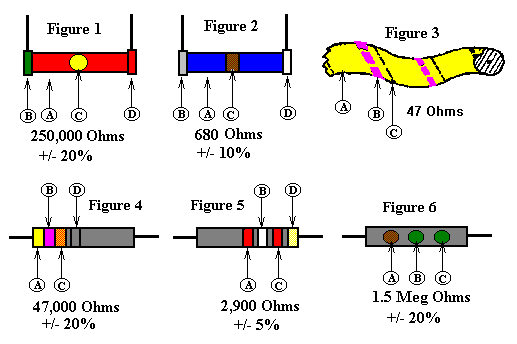A
First
Figure
B
Second
Figure
C
Number of zeros
after second figure
D
Tolerance
(+/-)
Black...0
Black...0
Black...none
Gold........5%
Brown...1
Brown...1
Brown...1
Silver.....10%
Red.....2
Red.....2
Red.....2
No Color...20%
Orange..3
Orange..3
Orange..3

Yellow..4
Yellow..4
Yellow..4

Green...5
Green...5
Green...5

Blue....6
Blue....6
Blue....6

Violet..7
Violet..7
Violet..7

Gray....8
Gray....8
Gray....8

White...9
White...9
White...9

Basis of the RMA Resistor Color Code System
The basis of the RMA Resistor Color code system is that all resistance values (in ohms) may be indicated by means of a system of three colors painted on the resistor in the form of narrow bands, dots, or as a body color. The percent of tolerance in the resistance value may be indicated by a fourth band of color.

Different manufactures adopted several different convenient arrangements for marking these color bands and dots on the resistors, but the same basis system applies to all of them. The system is as follows:

(1) the first figure of the resistance value in ohms is represented by one of the colors.
(2) the second figure is represented by another one of the colors.
(3) the number of zeros following the second figure is represented by the third color.
(4) the percent tolerance in the indicated resistance value is represented by the fourth color. Gold = 5%, Silver = 10%, and resistors rated at 20% bear no color bar.

Arrangements Used Commercially for Marking the Color Dots and Bands on Molded Fixed Resistors
Figures 1 and 2
(1) the first figure of the resistance value in ohms is indicated by the body color (A) of the resistor.
(2) the second figure is indicated by the color of one end (B) of the resistor.
(3) the number of zeros following the second figure is indicated by the color of a dot (C) --Figure 1, or a band (C) ---Figure 2, at the center of the resistor. When no center dot or band exists, it is to be assumed that the dot, or band is of the same color as the body and the number of zeros is judged from that color.
(4) The percent of tolerance of the resistance value is indicated by another colored band (D) (either gold or silver) at the other end of the resistor. In the absence of the tolerance band, the tolerance value is assumed to be +/- 20%.

Color Coding of Flexible Type Resistors
Flexible fabric-covered wire-wound resistors are also RMA coded by three identifying colors, the same as molded resistors. The method of identification is as follows:
Figure 3
(1) the body color (A) represents the first figure of the resistance value in ohms.
(2) the thickest thread (triple thread) color (B) represents the second figure.
(3) the thinnest thread (single-thread) color (C) represents the number of zeros following the two figures.
If either the thick or thin thread is missing, it is assumed to be there, carrying the same color as the body.

Figures 4 and 5
The consecutive colored band arrangements shown figures 4 and 5 are a later marking arrangement widely used on molded resistor--especially those provided with axial-type pigtail leads. In the marking arrangement shown in figure 4, the three (or four) bands are arranged side by side with band (A) indicating the first significant figure of the resistance value.
The band arrangement shown in figure 5 differs only in the position of the bands. Here, the three bands are located off-center on the resistor with the starting band (A) nearest the center. This really amounts to the same arrangement as figure 4, with the bands shifted to the right so that the tolerance band (D) is at the end. The band are read the same as figure 4, consecutively--left to right.

Figure 6
Some molded resistors look like small, narrow, mica capacitors. These are ordinarily RMA color-coded by means of three identifying colored dots placed as illustrated in Figure 6. These dots are read in sequence A-B-C, in the same manner as explained for the markings for Figure 4.

The letter K is normally used to designate Kilo (thousand ) ohms, and M for Meg (million) ohms. Example: 1K = 1,000 ohms -- 1.5M = 1,500,000 ohms. Be aware that on some early schematics, the letter M may sometimes be used to designate the value of 1000.
Why Some Radio Manufactures Employed Odd-Value Resistors
Have you ever wondered why manufactures use odd values of resistors? Values like 51,000 instead of 50,000, or 99,000 instead of 100,000.
Some of the larger manufactures--for example Philco--used mercury-vapor lighting in their assembly plants. Certain colors are difficult to distinguish under the bluish-green light of these lamps. To overcome this difficulty, these manufactures employed odd-values of resistors. For example, the black in the green-black-orange color of a 50,000-ohm resistor is not clearly distinguishable under this light. Therefore, instead of using a 50,000-ohm resistor, a 51,000-ohm unit was used instead, since each of the code colors (green-brown-green) for this value would show up sufficiently well. Likewise 99,000-ohm resistors were used in place of 100,000-ohm units, etc.
In practically all cases where such odd-value resistors are found in a set, the next higher or lower 'even' value can be satisfactorily substituted.